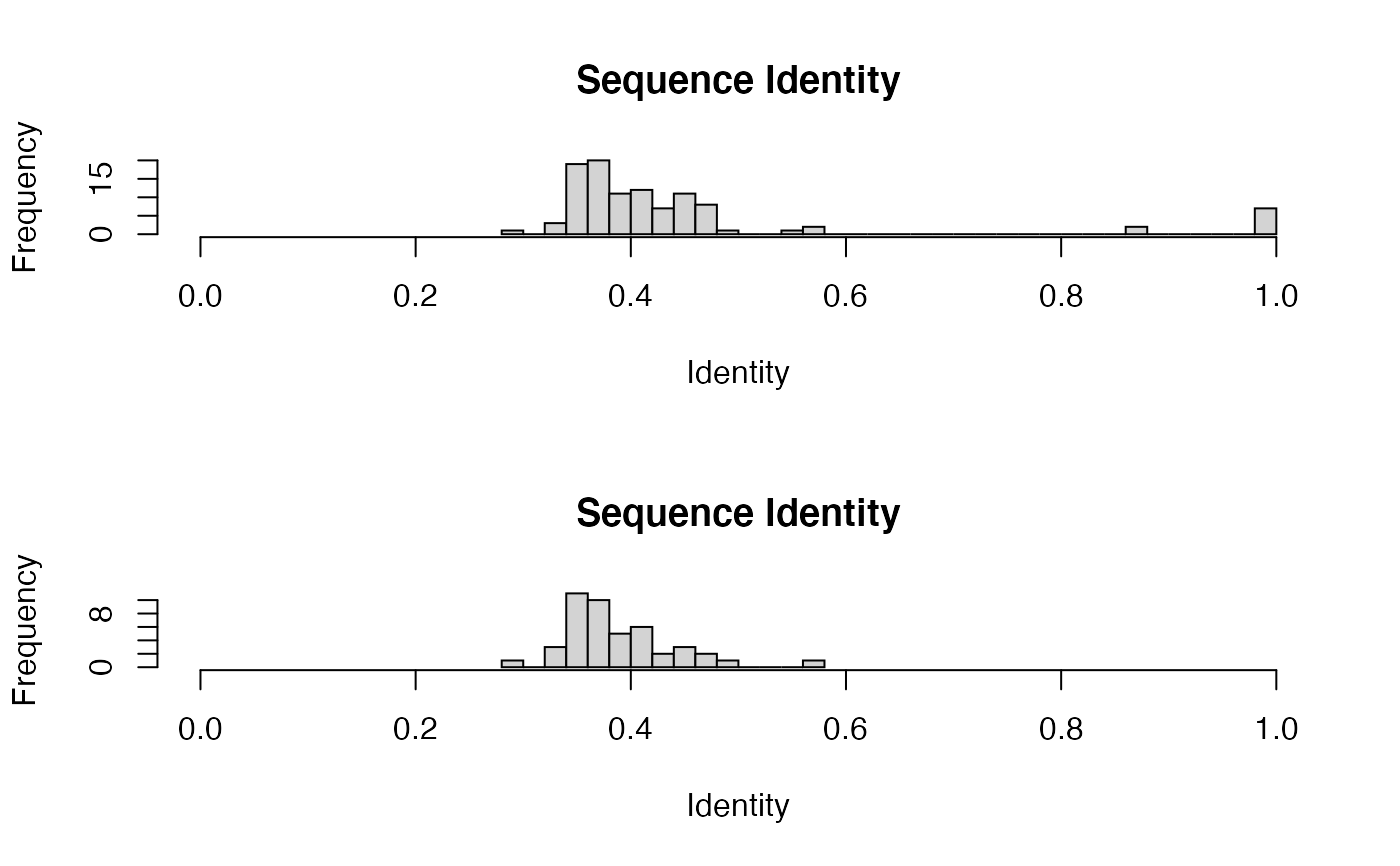Identify and filter subsets of sequences at a given sequence identity cutoff.

filter.identity(aln = NULL, ide = NULL, cutoff = 0.6, verbose = TRUE, ...)

## Arguments

aln sequence alignment list, obtained from seqaln or read.fasta, or an alignment character matrix. Not used if ‘ide’ is given. an optional identity matrix obtained from seqidentity. a numeric identity cutoff value ranging between 0 and 1. logical, if TRUE print details of the clustering process. additional arguments passed to and from functions.

## Details

This function performs hierarchical cluster analysis of a given sequence identity matrix ‘ide’, or the identity matrix calculated from a given alignment ‘aln’, to identify sequences that fall below a given identity cutoff value ‘cutoff’.

## Value

Returns a list object with components:

ind

indices of the sequences below the cutoff value.

tree

an object of class "hclust", which describes the tree produced by the clustering process.

ide

a numeric matrix with all pairwise identity values.

Grant, B.J. et al. (2006) Bioinformatics 22, 2695--2696.

## Author

Barry Grant

read.fasta, seqaln, seqidentity, entropy, consensus

## Examples

attach(kinesin)

ide.mat <- seqidentity(pdbs)

# Histogram of pairwise identity values
#> filter.identity(): N clusters @ cutoff =  10 ide.cut <- seqidentity(pdbs$ali[k$ind,])#plot(k$tree, axes = FALSE, ylab="Sequence Identity") #print(k$ind) # selected